# Calculus 2 : Integrals

## Example Questions

### Example Question #31 : Integrals

True or False: An (definite) integral is the same thing as an antiderivative.

False

True

False

Explanation:

This is not true. An integral of a function is defined to to be the limit of the area computed by a Riemann sum of a function as the number of rectangles used becomes large. An antiderivative of a function is simply a derivative done in reverse.

If you chose true, you were probably thinking of the fact that antiderivatives are used in evaluating (definite) integrals. That's what the Fundemental Theorem of Calculus tells us. That's not the only way of evaluating them though.

### Example Question #32 : Integrals

Assumeto be a continuous, integratabtle function.

Given the definite integrals: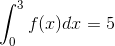and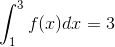.

Find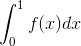.

-7

7

2

-2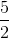2

Explanation:

Recall that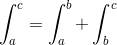assuming that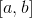and are adjacent intervals and the function being integrated is continuous and integratabtle.

For this problem one may create an equation: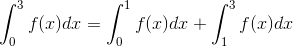Causing the equation to become: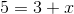Letting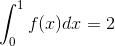### Example Question #32 : Integrals

Find: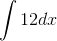.

UndefinedExplanation:

Evaluating the integral as a constant is the same as evaluating the integral: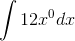, and if we use the Power Rule, we get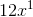, which can be simplified to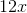.

### Example Question #31 : Integrals

What idea is related to integration?

Derivative

Implicit Differentiaton

Antiderivative

Integration

Antiderivative

Explanation:

Step 1: Recall the relationship between integration and other calculus topics..

Integration is the opposite of Differentiation
Differentiation is the opposite of Anti-Differentiation

Step 2: Using the relationships in step 1, we can clearly see:

Integration is the same as Anti-Differentiation..

The answer: Integration uses the idea of anti-derivatives.

### Example Question #1782 : Calculus Ii

Which of the following integrals is equivalent to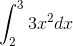?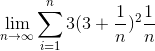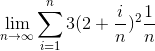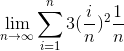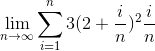None of the other answersExplanation:

The definition of integration relies on the equation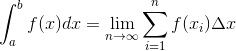, with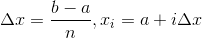.

For our given integral,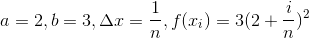. Substituting this into the right side of the equation and simplifying, we get.

### Example Question #34 : Integrals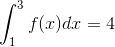and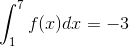.  What is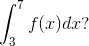Not enough information.Explanation:

If we think about integrals as the area under the curve from some value to another, then in the first integral, we are given one area.  The second integral from the problem statement gives us the entire region.  We are asked for the area under the curve for the between the two integrals.  Therefore, we need to subtract the areas given.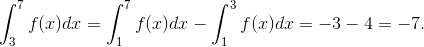### Example Question #32 : Integrals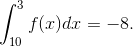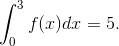What is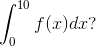Not enough information.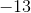Explanation:

Here, we want the total area under the curve fromto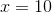.  Therefore, we need to add the other two parts that encompass the total area.  However, the first integral is given backwards (the lower integration limit is larger than the upper integration limit).  Whenever that occurs, we need to make that area negative: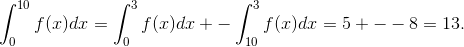### Example Question #1783 : Calculus Ii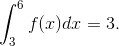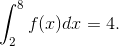What is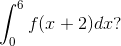Explanation:

Whenever we have transformations of integrals, we need to attempt to simplify the expression.  Because this transformation is a horizontal shift, we cannot split the integral.  However, if you look closely at the problem, you see that the limits of the integral have also changed.  The amount they have changed is the exact change of the transformation!  Therefore, the area under the curve of the function does not change.  This is a trick!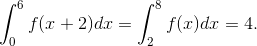### Example Question #37 : Integrals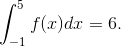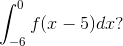Not enough information.Explanation:

Whenever we have transformations of integrals, we need to attempt to simplify the expression.  Because this transformation is a horizontal shift, we cannot split the integral.  However, if you look closely at the problem, you see that the limits of the integral have also changed.  The amount they have changed is the exact change of the transformation!  Therefore, the area under the curve of the function does not change.  This is a trick!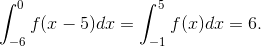### Example Question #36 : Integrals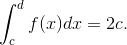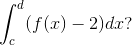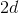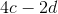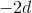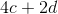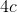Explanation:

For this problem, we need to first simplify the integral by splitting in two integrals.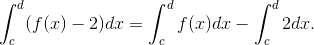Since, the second integral is negative, I just pulled the constant outside the integral and made it subtraction.  The first integral has been given to us in the problem.  Therefore, all we need to do is evaluate the second integral, and subtract that from the first.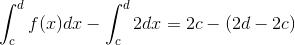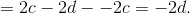### All Calculus 2 Resources